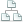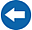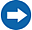Home >Tips >Excel 2016 from Scratch >Bind Datasheets BSite map

# Excel 2016 from Scratch Exercise Bind Datasheets B

## An Excel tutorial by Peter KalmstromIn this demo in the Excel 2016 from Scratch series, Peter Kalmstrom shows how to fetch values from one Excel sheet to a formula in another sheet, so that changes in the source sheet will reflect in the other sheet.

As an example Peter uses production prices for different kinds of ice creams, but this way of fetching info from other sheets can of course be used for any calculation.

Exercise
If you want to try the exercise yourself, please download the Excel file Peter uses in the demo!

Now Peter is moving to a bit more complex calculation than he has shown before in this Excel series.

### Content

This is what Peter shows in the demo below:
• How to calculate the sum of all ingredients costs for a batch by using the AutoSum button.
• How to calculate the production price of one ice-cream by using a formula that counts division.
• How to change the number format into dollars.
• How to create a formula to add a value from one sheet into another sheet.
• How to copy a format from one cell to another.
Peter uses Excel 2016 for his demo, but the Excel basics are the same for earlier versions of Excel.